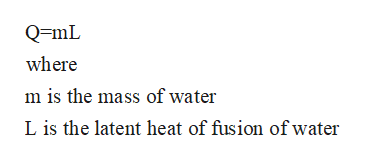# Given that a 10.0g sample of ice at 0.0 degrees celsius melts and then the resultant water proceeds to warm to 20.0 degrees celsius, there are two separate processes (dealing with the transfer of heat) that describe the occurence. What are those two processes? (Hint one involves the specific heat of water).  Determine the delta H of the following reactions:CH4 (g) +2O2(g) → CO2 (g) +2H2O(l)CH4 (g) +2O2(g) → CO2 (g) +2H2O(g) What is the numerical difference between these two values?What is the only physical difference between the two reactions?What does the numerical difference represent, in terms of the physical difference between the two reactions? A 4.00g sample of rocket fuel was combusted in a bomb calorimeter according to the following reaction:2CH6N2(l)+ 5O2(g) →  2N2(g) +2CO2(g) + 6H2O(l) The temperature of the surrounding calorimeter increased from 25.00C to 39.50 C and the heat capacity of the calorimeter was previously determined to be 7.794KJ/c. What is the heat of the reaction for one mole of rocket fuel, CH6,N2?

Question
55 views

•

Given that a 10.0g sample of ice at 0.0 degrees celsius melts and then the resultant water proceeds to warm to 20.0 degrees celsius, there are two separate processes (dealing with the transfer of heat) that describe the occurence. What are those two processes? (Hint one involves the specific heat of water).

1. Determine the delta H of the following reactions:

CH4 (g) +2O2(g) → CO2 (g) +2H2O(l)

CH4 (g) +2O2(g) → CO2 (g) +2H2O(g)

1. What is the numerical difference between these two values?
2. What is the only physical difference between the two reactions?
3. What does the numerical difference represent, in terms of the physical difference between the two reactions?

A 4.00g sample of rocket fuel was combusted in a bomb calorimeter according to the following reaction:

2CH6N2(l)+ 5O2(g) →  2N2(g) +2CO2(g) + 6H2O(l)

The temperature of the surrounding calorimeter increased from 25.00C to 39.50 C and the heat capacity of the calorimeter was previously determined to be 7.794KJ/c. What is the heat of the reaction for one mole of rocket fuel, CH6,N2?

check_circle

Step 1

Note: As per our code, we are allowed to answer only the first question when a collection of questions are provided to us. Thus, the answer to your first question is as follows:

When 10 g of ice melts at 0°C to form water at 0°C, the heat provided is the latent heat of fusion of water. This is the heat that gives the required kinetic energy to the water molecules to overcome the intermolecular forces that are binding it in the solid from. The value for the heat of fusion of ice is 334 J/g.

Step 2

When the ice has melted then it is taken to the temperature of 20°C. here the specific heat involved is the specific heat capacity of water which is the heat required to raise the temperature of unit mass of water by unit degree increase in temperature. The value of specific heat of water is 4.182 J/kg°C.

Step 3

The total heat involved in raising the temperature ...help_outlineImage TranscriptioncloseQmL where m is the mass of water L is the latent heat of fusion of water fullscreen

### Want to see the full answer?

See Solution

#### Want to see this answer and more?

Solutions are written by subject experts who are available 24/7. Questions are typically answered within 1 hour.*

See Solution
*Response times may vary by subject and question.
Tagged in

### Chemistry